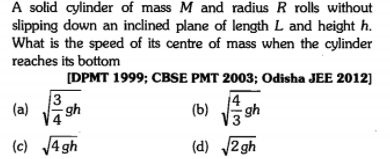Question 20 of 35A solid cylinder of mass M and radius R rolls without slipping down an inclined plane of length L and height h. What is the speed of its centre of mass when the cylinder reaches its bottom.

(a) $\sqrt{\frac{3}{4}\mathrm{gh}}$                                        (b) $\sqrt{\frac{4}{3}\mathrm{gh}}$

(c)                                          (d)